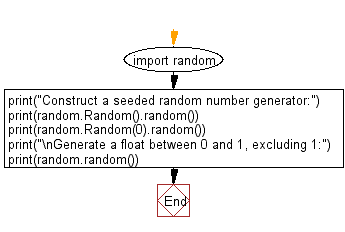﻿ Python: Construct a seeded random number and float number generator - w3resource# Python: Construct a seeded random number and float number generator

## Python module: Exercise-4 with Solution

Write a Python program to construct a seeded random number generator, also generate a float between 0 and 1, excluding 1.

Use random.random()

Sample Solution:

Python Code:

``````import random
print("Construct a seeded random number generator:")
print(random.Random().random())
print(random.Random(0).random())
print("\nGenerate a float between 0 and 1, excluding 1:")
print(random.random())
```
```

Sample Output:

```Construct a seeded random number generator:
0.4209937412831368
0.8444218515250481

Generate a float between 0 and 1, excluding 1:
0.5000494615698478
```

Flowchart:## Visualize Python code execution:

The following tool visualize what the computer is doing step-by-step as it executes the said program:

Python Code Editor:

Have another way to solve this solution? Contribute your code (and comments) through Disqus.

What is the difficulty level of this exercise?

Test your Python skills with w3resource's quiz

﻿

## Python: Tips of the Day

For-else construct useful when searched for something and find it:

```# For example assume that I need to search through a list and process each item until a flag item is found and
# then stop processing. If the flag item is missing then an exception needs to be raised.

for i in mylist:
if i == theflag:
break
process(i)
else:
raise ValueError("List argument missing terminal flag.")
```